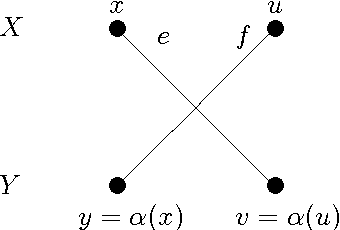You are currently offline. Some features of the site may not work correctly.

# Digraph realization problem

The digraph realization problem is a decision problem in graph theory. Given pairs of nonnegative integers , the problem asks whether there is a… Expand
Wikipedia

## Papers overview

Semantic Scholar uses AI to extract papers important to this topic.
2014
2014
Abstract Given pairs ( a 1 , b 1 ) , … , ( a n , b n ) of nonnegative integers, the digraph realization problem for digraphs asks… Expand
•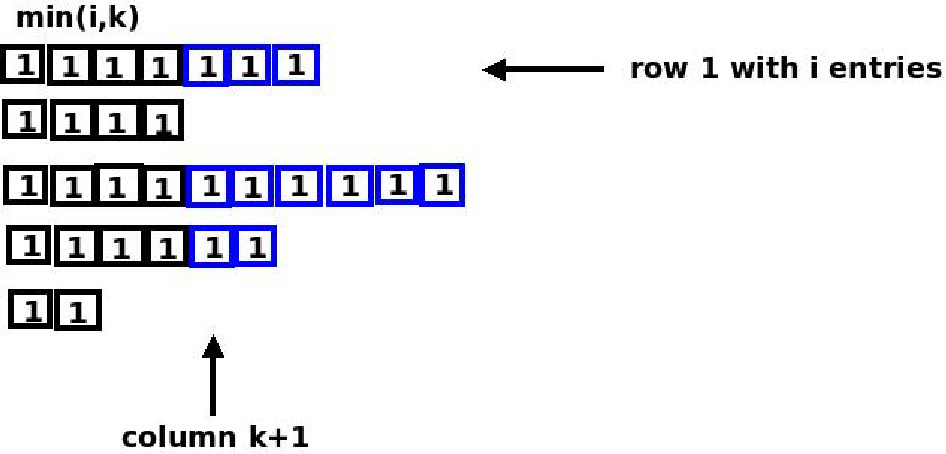2012
2012
• SEA
• 2012
• Corpus ID: 14611614
We study the following fundamental realization problem of directed acyclic graphs (dags). Given a sequence $S:={a_1 \choose b_1… Expand •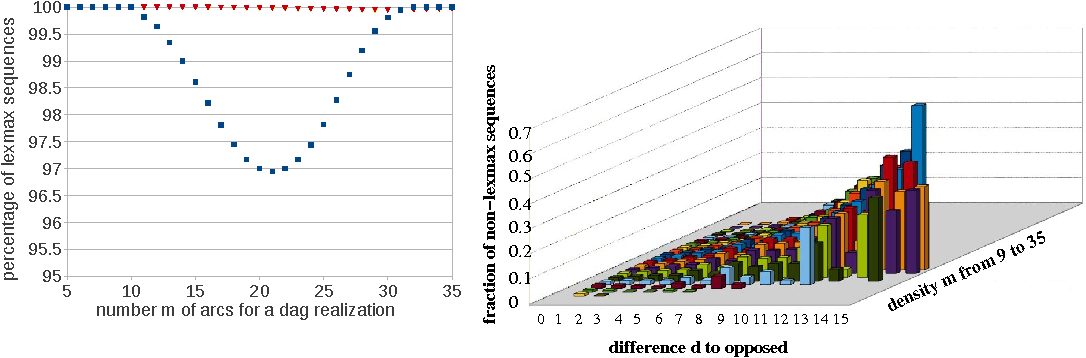•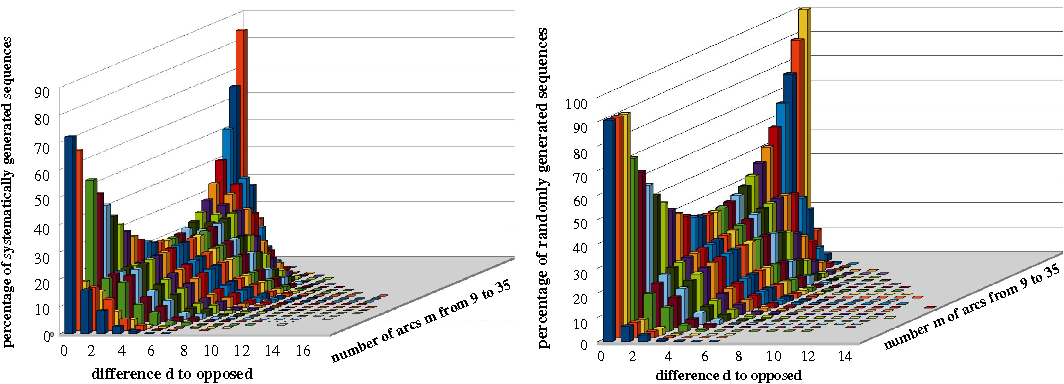•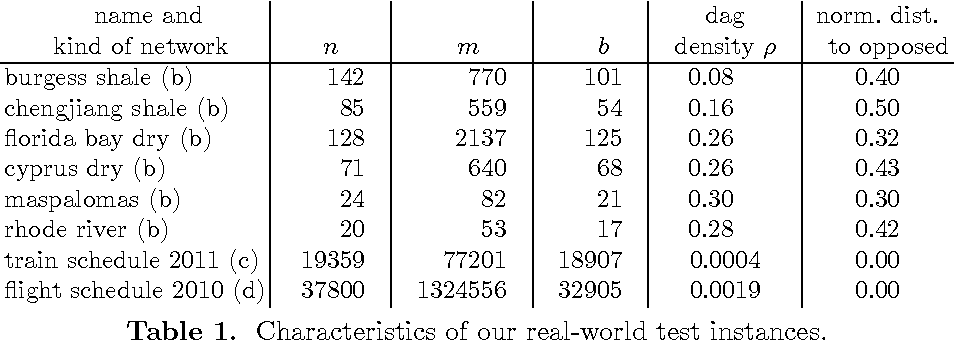•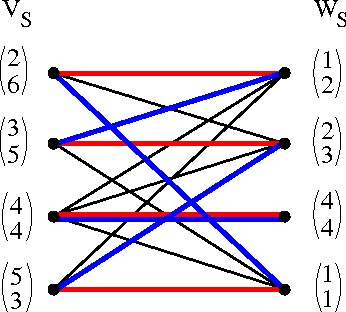•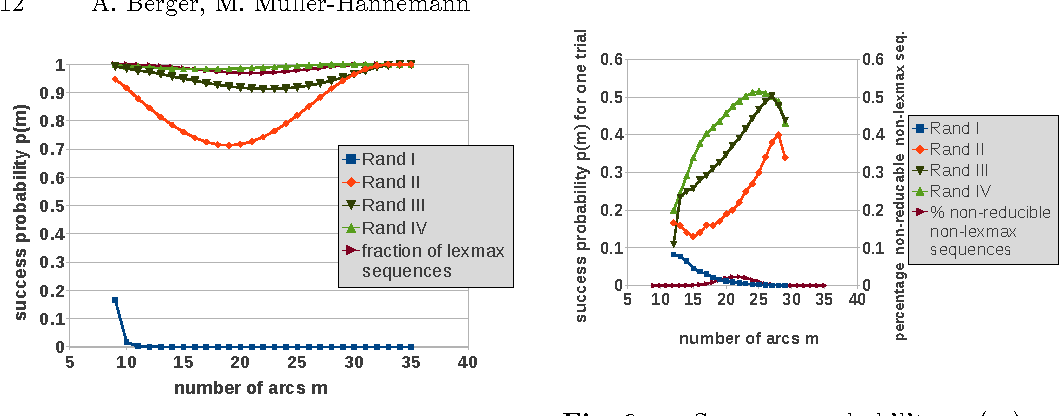2012 2012 The \emph{graph realization problem} is to find for given nonnegative integers$a_1,\dots,a_n\$ a simple graph (no loops or… Expand
2009
2009
• Discret. Appl. Math.
• 2009
• Corpus ID: 5858053
Given a digraph D, the set of all pairs (N^-(v),N^+(v)) constitutes the neighborhood dihypergraph N(D) of D. The Digraph… Expand
•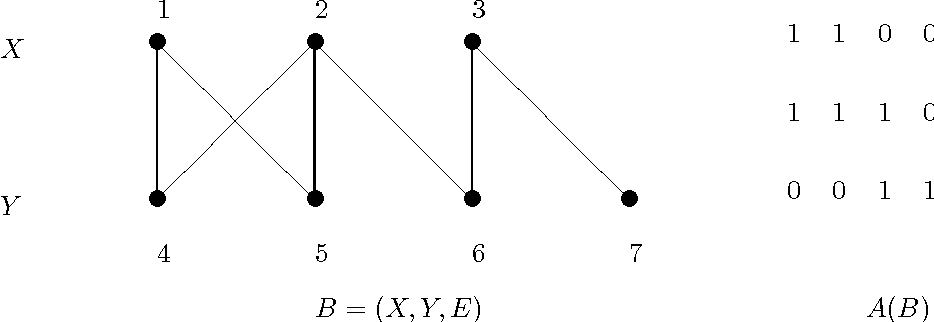•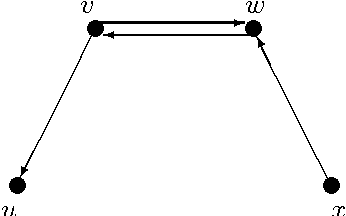•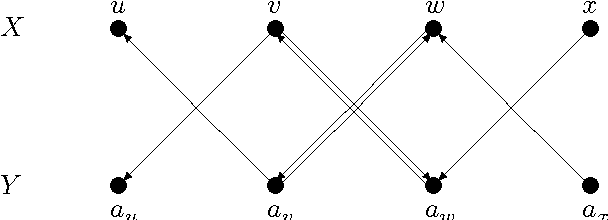•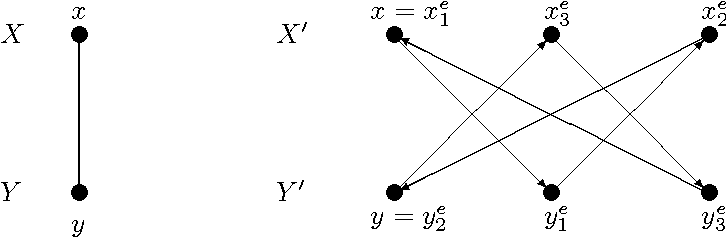•